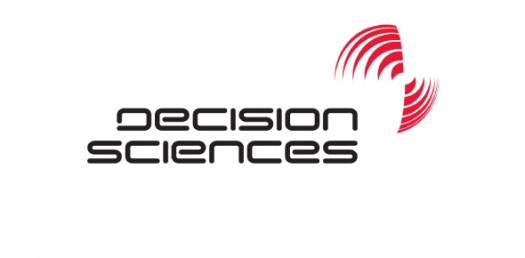# Decision Sciences Trivia Quiz

20 Questions | Attempts: 680SettingsDecision science is a toolbox of approaches and you learn which approach to take when you face certain problem. Economic rationality involves decision-making where individuals have to decide on which need to satisfy first. How well do you understand decision science? Take the quick test to find out!

• 1.
Which of the following is a valid objective function for a linear programming problem?
• A.

Min 4X + 3Y + 6Z

• B.

Max 5 XY

• C.

Max 5X2 + 6Y4

• D.

Min (X1+X2) / 3

• 2.
A simulation model uses the mathematical expressions and logical relationships of the –
• A.

Computer Model

• B.

Performance Measures

• C.

Real system

• D.

Estimated inferences

• 3.
The maximization or minimization of a quantity is the -
• A.

Goal of a management science

• B.

Objective of linear programming

• C.

Decision for decision analysis

• D.

Constraint of operation research

• 4.
The manner in which units receive their service, such as FCFS, is the –
• A.

Operating Characteristics

• B.

• C.

Channel

• D.

Queue Discipline

• 5.
The solution of a transportation problem, with m- rows ( supplies) and n – columns ( destinations) is feasible if the number of occupied cells are –
• A.

M + n -1

• B.

M + n

• C.

M X n

• D.

M + n -1

• 6.
Customer behavior in which he moves from one queue to another in multiple channel situation is –
• A.

Reneging

• B.

Backing

• C.

Jockeying

• D.

Alternating

• 7.
In the queue model notation (a/b/c) : (d/e), what does c represent –
• A.

Arrival pattern

• B.

Number of services

• C.

Service pattern

• D.

Capacity of the system

• 8.
The prohibited cell in transportation problem is considered by considering its cost –
• A.

0

• B.

Negative

• C.

- ∞

• D.

• 9.
The dummy source or destinations in a transportation problem is added to –
• A.

Satisfy the rim conditions

• B.

Present solution from becoming degenerate

• C.

Ensure that the total cost does not exceed a limit

• D.

None of the above

• 10.
Analysis of a Markov Process -
• A.

Optimizes the system

• B.

Describes future behavior of the system

• C.

Leads to higher order decision making

• D.

All of the alternatives are true.

• 11.
In a multiple channel system –
• A.

µ > λ

• B.

Each server has its own queue

• C.

Each server has the same service rate

• D.

All of the alternatives are correct

• 12.
In case a worker can not assigned a particular job –
• A.

The relevant cost item is replaced by a zero

• B.

The relevant cost item is the same as the lowest cost in the entire matrix

• C.

The relevant cot item is replaced by M a very large number

• D.

The relevant cost item is subtracted from all the other cost item

• 13.
Utilization factor is –
• A.

Service rate divided by arrival rate

• B.

Length in the system divided by length in the queue

• C.

Waiting time in system divided by waiting time in queue

• D.

Arrival rate divided by service rate

• 14.
In (M/M/1) : (∞/FCFS) model, the system length  Ls is given by -
• A.

ϱ / 1-ϱ

• B.

λ 2 / µ - λ

• C.

1/ µ - λ

• D.

ρ2 / 1-ρ

• 15.
Assignment problem is solved by –
• A.

Simplex Method

• B.

Graphical Method

• C.

Vector Method

• D.

Hungarian Method

• 16.
If Ro represent state probabilities of present period then the state probabilities end of second period is given by –
• A.

R2 = R1 X P2

• B.

R2 = (R0 X P2) X (R0XP2)

• C.

R2 = P X R1

• D.

None of the above

• 17.
The property of the optimum assignment matrix is –
• A.

It will not have zero as its element.

• B.

It will have zero as elements of one diagonal

• C.

It will have zero at least one zero in each row and column

• D.

None

• 18.
Which of the following is not an assumption of the single server queuing method –
• A.

Mean arrival rate < mean service rate

• B.

Queue discipline is not first came first serve

• C.

• D.

Service times are Poisson distributed

• 19.
In Monte Carlo Simulation , the solutions obtained using different set of random numbers will be –
• A.

Different

• B.

Exactly same

• C.

Non-real

• D.

None of the above

• 20.
The first step in solving operations research problem is -
• A.

Model building

• B.

Obtain alternate solutions

• C.

Interpreting the variables

• D.

Formulation of the problem

## Related TopicsBack to top
×

Wait!
Here's an interesting quiz for you.